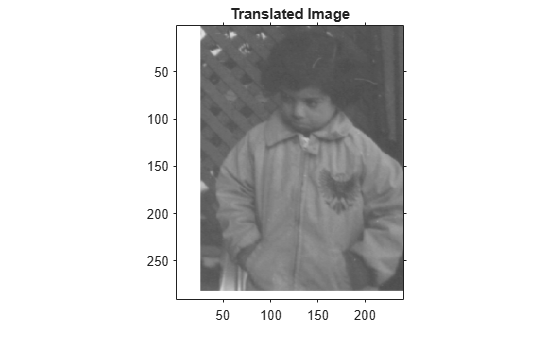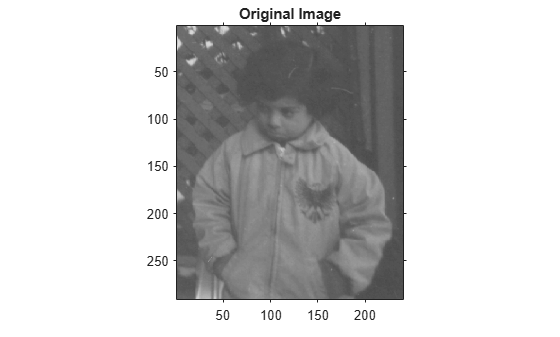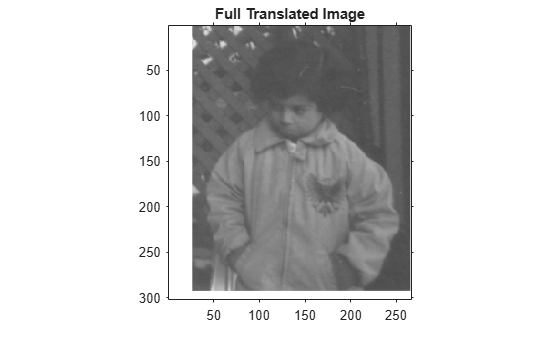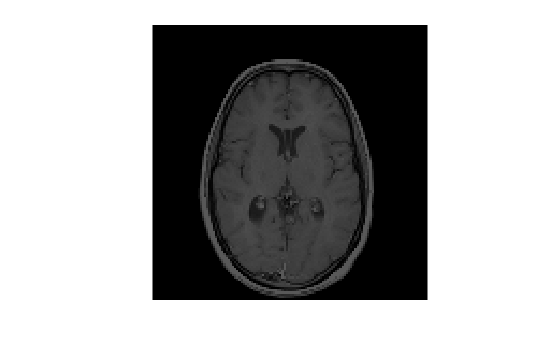# imtranslate

Translate image

## Syntax

``B = imtranslate(A,translation)``
``````[B,RB] = imtranslate(A,RA,translation)``````
``___ = imtranslate(___,method)``
``___ = imtranslate(___,Name,Value)``

## Description

example

````B = imtranslate(A,translation)` translates image `A` by the 2-D or 3-D translation vector specified in `translation`.If `A` has more than two dimensions and `translation` is a 2-element vector, then `imtranslate` applies the 2-D translation to each plane of `A`.```
``````[B,RB] = imtranslate(A,RA,translation)``` translates the spatially referenced image `A` with its associated spatial referencing object `RA`. The translation vector, `translation`, is in the world coordinate system. The function returns the translated spatially referenced image `B`, with its associated spatial referencing object, `RB`.```
````___ = imtranslate(___,method)` translates image `A`, using the interpolation method specified by `method`.```

example

````___ = imtranslate(___,Name,Value)` translates the input image using name-value pairs to control various aspects of the translation.```

## Examples

collapse all

`I = imread('pout.tif');`

Translate the image.

`J = imtranslate(I,[25.3, -10.1],'FillValues',255);`

Display the original image and the translated image.

```figure imshow(I); title('Original Image'); set(gca,'Visible','on');``````figure imshow(J); title('Translated Image'); set(gca,'Visible','on');````I = imread('pout.tif');`

Translate the image. Use the `OutputView` parameter to specify that you want the entire translated image to be visible.

`J = imtranslate(I,[25.3, -10.1],'FillValues',255,'OutputView','full');`

Display the original image and the translated image.

```figure imshow(I); title('Original Image'); set(gca,'Visible','on');``````figure imshow(J); title('Full Translated Image'); set(gca,'Visible','on');```Load MRI data into the workspace and display it.

```s = load('mri'); mriVolume = squeeze(s.D); sizeIn = size(mriVolume); hFigOriginal = figure; hAxOriginal = axes; slice(double(mriVolume),sizeIn(2)/2,sizeIn(1)/2,sizeIn(3)/2); grid on, shading interp, colormap gray```Apply a translation in the X,Y direction.

`mriVolumeTranslated = imtranslate(mriVolume,[40,30,0],'OutputView','full');`

Visualize the translation by viewing an axial slice plane taken through center of the volume. Note the shift in the X and Y directions.

```sliceIndex = round(sizeIn(3)/2); axialSliceOriginal = mriVolume(:,:,sliceIndex); axialSliceTranslated = mriVolumeTranslated(:,:,sliceIndex); imshow(axialSliceOriginal);````imshow(axialSliceTranslated);`## Input Arguments

collapse all

Image to be translated, specified as a numeric array, logical array, or categorical array.

Data Types: `single` | `double` | `int8` | `int16` | `int32` | `uint8` | `uint16` | `uint32` | `logical` | `categorical`

Spatial referencing information associated with the input image `A`, specified as an `imref2d` or `imref3d` spatial referencing object.

Translation vector, specified as a 2-element numeric vector `[Tx Ty]` or a 3-element numeric vector `[Tx Ty Tz]`. Values can be fractional.

Data Types: `single` | `double` | `int8` | `int16` | `int32` | `uint8` | `uint16` | `uint32`

Interpolation method, specified by one of the following values.

Value

Description

`'nearest'`

Nearest-neighbor interpolation. The output pixel is assigned the value of the pixel that the point falls within. No other pixels are considered.

Nearest-neighbor interpolation is the only method supported for categorical images and it is the default method for images of this type.

`'bilinear'`

Linear interpolation.

Linear interpolation is the default method for numeric and logical images.

`'bicubic'`

Cubic interpolation.

Note

Cubic interpolation can produce pixel values outside the original range.

Data Types: `char` | `string`

### Name-Value Arguments

Specify optional pairs of arguments as `Name1=Value1,...,NameN=ValueN`, where `Name` is the argument name and `Value` is the corresponding value. Name-value arguments must appear after other arguments, but the order of the pairs does not matter.

Before R2021a, use commas to separate each name and value, and enclose `Name` in quotes.

Example: `mriVolumeTranslated = imtranslate(mriVolume,[40,30,0],'OutputView','full');`

Output world limits, specified as the comma-separated pair consisting of `'OutputView'` and one of the following values.

ValueDescription
`'same'`Output world limits are the same as the input image.
`'full'`Output world limits are the bounding rectangle that includes both the input image and the translated output image.

Data Types: `char` | `string`

Fill values used for output pixels outside the input image, specified as the comma-separated pair consisting of `'FillValues'` and one of the following values. `imtranslate` uses fill values for output pixels when the corresponding inverse transformed location in the input image is completely outside the input image boundaries.

The default fill value of numeric and logical images is `0`. The default fill value of categorical images is `missing`, which corresponds to the `<undefined>` category.

Image Type

Translation Dimension

Format of Fill Values

2-D grayscale or logical image2-D
• Numeric scalar

2-D color image or 2-D multispectral image2-D
• Numeric scalar

• c-element numeric vector specifying a fill value for each of the c channels. The number of channels, c, is 3 for color images.

Series of p 2-D images2-D

• Numeric scalar

• c-by-p numeric matrix. The number of channels, c, is 1 for grayscale images and 3 for color images.

N-D image2-D
• Numeric scalar

• Numeric array whose size matches dimensions 3-to-N of the input image `A`. For example, if `A` is 200-by-200-by-10-by-3, then `FillValues` can be a 10-by-3 array.

3-D grayscale or logical image3-D
• Numeric scalar

Categorical image2-D or 3-D
• Valid category in the image, specified as a string scalar or character vector.

• `missing`, which corresponds to the `<undefined>` category. For more information, see `missing`.

Example: `255` fills a `uint8` image with white pixels

Example: `1` fills a `double` image with white pixels

Example: `[0 1 0]` fills a `double` color image with green pixels

Example: `[0 1 0; 0 1 1]'`, for a series of two `double` color images, fills the first image with green pixels and the second image with cyan pixels

Example: `"vehicle"` fills a categorical image with the "vehicle" category

Data Types: `single` | `double` | `int8` | `int16` | `int32` | `int64` | `uint8` | `uint16` | `uint32` | `uint64` | `string` | `char`

## Output Arguments

collapse all

Translated image, returned as a numeric, logical, or categorical array of the same data type as the input image, `A`.

Spatial referencing information associated with the output image, returned as an `imref2d` or `imref3d` spatial referencing object.

## Tips

• `imtranslate` is optimized for integrally valued `translation` vectors.

• When `'OutputView'` is `'full'` and `translation` is a fractional number of pixels, then `imtranslate` expands the world limits of the output spatial referencing object to the nearest full pixel increment. `imtranslate` does this so that it contains both the original and translated images at the same resolution as the input image. The additional image extent in each is added on one side of the image, in the direction that the translation vector points. For example, when `translation` is fractional and positive in both X and Y, then `imtranslate` expands the maximum of `XWorldLimits` and `YWorldLimits` to enclose the `'full'` bounding rectangle at the resolution of the input image.

## Version History

Introduced in R2014a